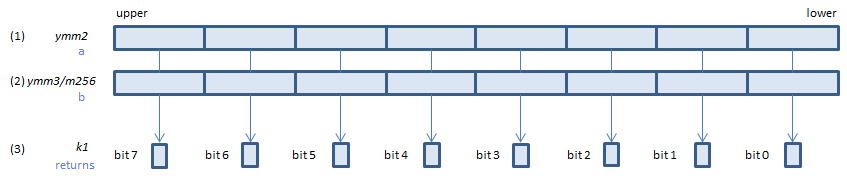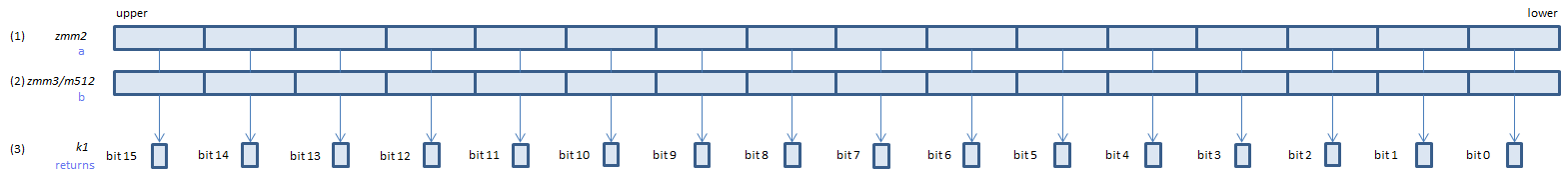﻿ pcmpeqd

## PCMPEQD - Packed CoMPare EQual Dword

PCMPEQD xmm1, xmm2/m128    (S2
__m128i _mm_cmpeq_epi32(__m128i a, __m128i b)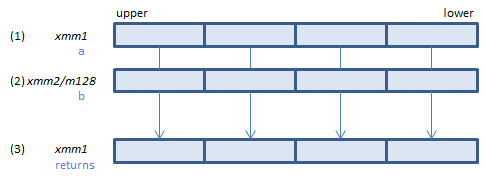For each DWORD, if (1) == (2) set 1, else set 0, to all bits in the corresponding DWORD of (3).
VPCMPEQD xmm1, xmm2, xmm3/m128    (V1
__m128i _mm_cmpeq_epi32(__m128i a, __m128i b)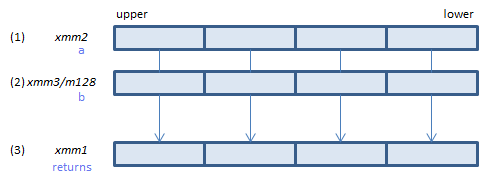For each DWORD, if (1) == (2) set 1, else set 0, to all bits in the corresponding DWORD of (3).
VPCMPEQD ymm1, ymm2, ymm3/m256    (V2
__m256i _mm256_cmpeq_epi32(__m256i a, __m256i b)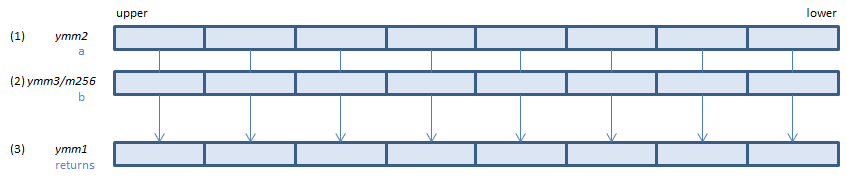For each DWORD, if (1) == (2) set 1, else set 0, to all bits in the corresponding DWORD of (3).
VPCMPEQD k1{k2}, xmm2, xmm3/m128/m32bcst    (V5+VL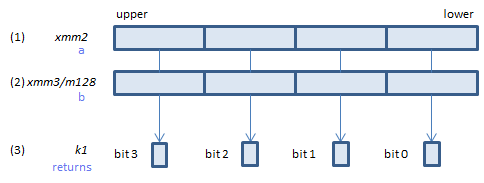For each DWORD, if (1) == (2) set 1, else set 0, to the corresponding bit of (3).
If k2 bit is 0, the comparison is not done and the corresponding bit of (3) is set to zero. Upper bits of (3) are zero cleared.
VPCMPEQD k1{k2}, ymm2, ymm3/m256/m32bcst    (V5+VL